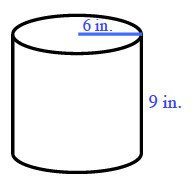### Home > CCG > Chapter 9 > Lesson 9.1.4 > Problem9-46

9-46.

Draw a cylinder on your paper. Assume the radius of the cylinder is $6$ inches and the height is $9$ inches.

1. What is the surface area of the cylinder? What is the volume?

The surface area of a cylinder is the sum of the areas of its top base, its bottom base, and its lateral surface.

$\text{Surface area of top base} = 36\pi \; \text{in.}^2$

$\text{Surface area of bottom base} = \text{Surface area of top base}$

$\text{Area of lateral surface} = \left(12\pi\right)\left(9\right) = 108\pi \; \text{in.}$$\text{SA} = 2 \left(36π\right) + 108π = 180π \; \text{in.}^2$

$\text{V} = 9\left(36\pi\right) = 324\pi \approx 1,017.9 \; \text{in.}^3$

2. If the cylinder is enlarged with a linear scale factor of $3$, what is the volume of the enlarged cylinder? How do you know?

How does increasing the scale by $3$ affect the volume? What must you multiply the volume by to determine the new volume?